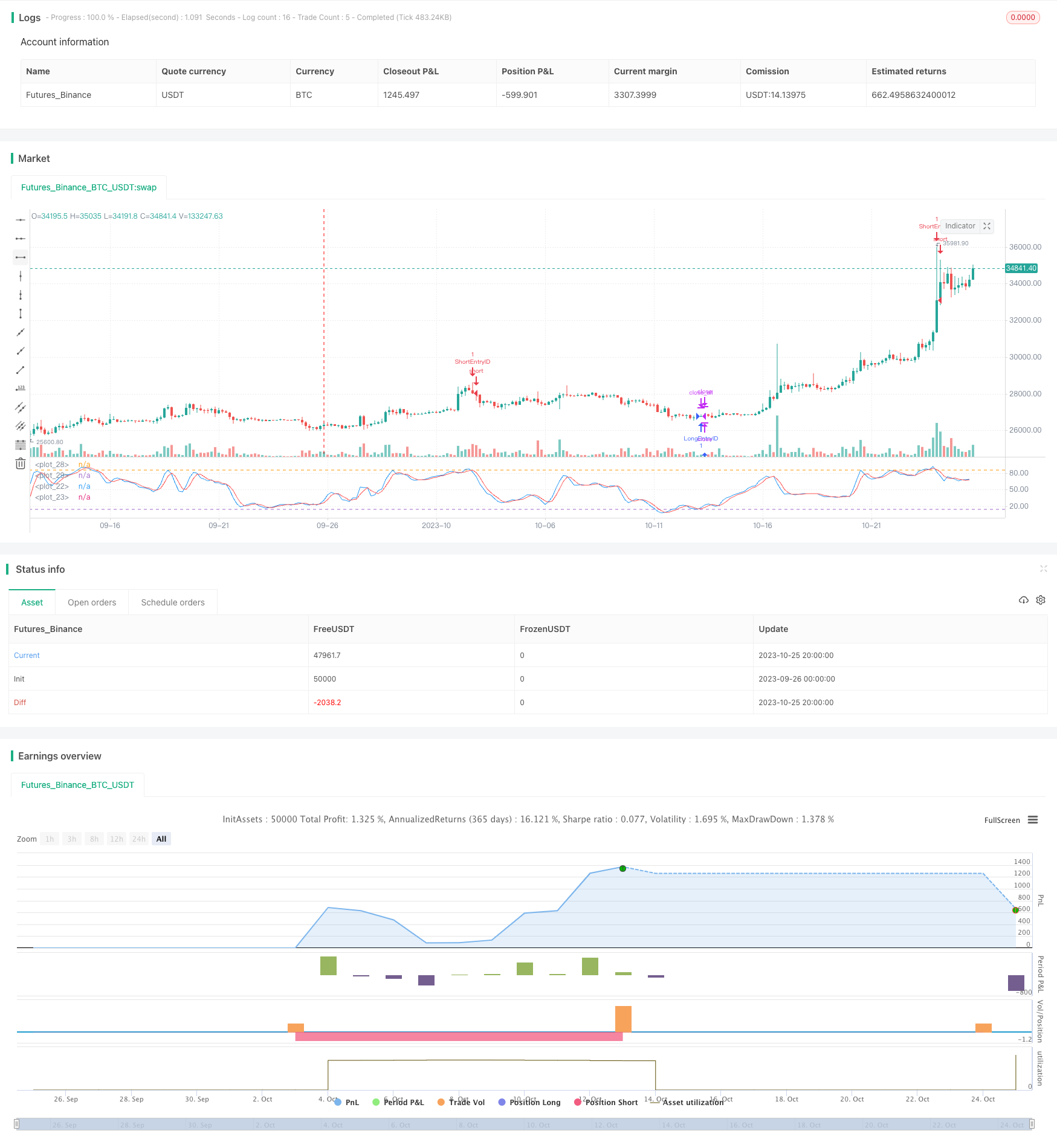# Classic Dual Moving Average Crossover Strategy

Author: ChaoZhang, Date: 2023-10-27 16:47:30
Tags:## Overview

The dual moving average crossover strategy is a very classic and commonly used technical analysis strategy. This strategy utilizes the crossover of a faster moving average and a slower moving average as the trading signals for buying and selling. When the faster moving average crosses above the slower moving average from below, a buy signal is generated. When the faster moving average crosses below the slower moving average from above, a sell signal is generated.

## Strategy Logic

The key parts of the strategy code include:

1. Define the length and type of fast and slow moving averages: the fast MA has a period of 5, the slow MA has a period of 21, both using simple moving average.

2. Calculate the fast and slow MAs: using the sma function to compute the 5-period and 21-period simple moving averages.

3. Plot the chart: plot the trend lines of the fast and slow MAs.

4. Define the entry and exit rules: buy when the fast MA crosses above the slow MA, sell when the fast MA crosses below the slow MA.

5. Execute trades: use the strategy’s long and short functions to automatically execute trades when conditions are met.

The key of this strategy is using moving averages of different periods to form the fast and slow MAs, and using their crossovers as trading signals. The fast MA captures price changes faster while the slow MA reflects long term trend better. The crossover of the fast MA above the slow MA indicates an upside breakout, which is a buy signal. And the crossover below is a sell signal. The logic of this strategy is simple and easy to implement.

The dual moving average crossover strategy has the following advantages:

1. Simple principle, easy to grasp, suitable for beginners.

2. Follow the price trend, small pullback.

4. Customizable parameters, flexible to adapt to market changes.

5. Easy to optimize and find suitable personal parameter sets.

6. Can set stop loss to control risk.

7. Can be used in various markets, high applicability.

8. Can be combined with other indicators to improve performance.

## Risk Analysis

There are also some risks with this strategy:

1. Delayed reaction when the trend is strong, could miss best entry timing. Can shorten the MA periods to improve sensitivity.

2. More false signals during range-bound markets. Can add filters to avoid wrong trades.

3. Too many trades can impact profitability. Can widen the MA distance to reduce crossovers.

4. Hard to determine trend, risk of counter-trend trading. Can add trend indictors.

5. Parameter optimization requires sufficient historical data, risk of overfitting with new products. Need to test robustness of parameters.

6. Single indicator susceptible to external factors, performance could be unstable. Can combine with other indicators for verification.

## Optimization Directions

There are some ways to optimize the dual MA strategy further:

1. Test different fast and slow MA lengths to find the optimal parameters for specific trading products.

2. Add filters like trading volumes, ATR stop loss to reduce inferior opportunities.

3. Combine momentum indicators to confirm trading signals and avoid false breakouts.

4. Optimize stop loss strategies to avoid premature or late exits.

5. Incorporate trend and wave indicators to enable trend following and counter-trend trading.

6. Use adaptive MAs to adjust parameters based on market conditions rather than fixed periods.

7. Utilize combinations of parameters for different market sessions and characteristics.

8. Perform real-time optimization via machine learning algorithms to continuously improve parameters.

## Summary

With its simple logic and ease of implementation, the dual moving average crossover strategy has become one of the most essential and widely used technical analysis strategies. It follows the price trend with controlled pullback and acceptable risk. But there is also huge potential for optimization, by parameter tuning, incorporating other indicators and automated algorithms, its applicability and performance can be further enhanced. Overall, the dual MA crossover strategy deserves great attention and long-term application by investors.

```/*backtest
start: 2023-09-26 00:00:00
end: 2023-10-26 00:00:00
period: 4h
basePeriod: 15m
exchanges: [{"eid":"Futures_Binance","currency":"BTC_USDT"}]
*/

//@version=3
// strategy("Stochastic Strategy of BiznesFilosof", shorttitle="SS of BiznesFilosof", overlay=false, initial_capital=10000, default_qty_type=strategy.percent_of_equity, default_qty_value=20, commission_type=strategy.commission.percent, commission_value=0.15, pyramiding=0)

//Period
startY = input(title="Start Year", defval = 2011)
startM = input(title="Start Month", defval = 1, minval = 1, maxval = 12)
startD = input(title="Start Day", defval = 1, minval = 1, maxval = 31)
finishY = input(title="Finish Year", defval = 2050)
finishM = input(title="Finish Month", defval = 12, minval = 1, maxval = 12)
finishD = input(title="Finish Day", defval = 31, minval = 1, maxval = 31)
//finish = input(2019, 02, 28, 00, 00)
timestart = timestamp(startY, startM, startD, 00, 00)
timefinish = timestamp(finishY, finishM, finishD, 23, 59)
window = true // Lenghth strategy

length1 = input(21, minval=1), smoothK1 = input(3, minval=1), smoothD1 = input(3, minval=1)
//length2 = input(5, minval=1), smoothK2 = input(1, minval=1), smoothD2 = input(1, minval=1)
inh0 = input(title="Bottom Line", defval = 14, minval=0), inh1 = input(title="Upper Line", defval = 86, minval=0)

k1 = sma(stoch(close, high, low, length1), smoothK1)
d1 = sma(k1, smoothD1)
plot(k1, color=blue)
plot(d1, color=red)
//k2 = sma(stoch(close, high, low, length2), smoothK2)
//d2 = sma(k2, smoothD2)
//plot(k2, color=orange)

h1 = hline(inh1)
h0 = hline(inh0)
fill(h0, h1, color = aqua, transp=90)

//open
strategy.entry("LongEntryID", strategy.long, comment="LONG", when = crossover(k1, d1) and crossover(k1, inh0) and window)
strategy.entry("ShortEntryID", strategy.short, comment="SHORT", when = crossunder(k1, d1) and crossunder(k1, inh1) and window)

if crossunder(k1, d1) and crossunder(k1, inh1) and strategy.position_size > 0
strategy.close_all()
if crossover(k1, d1) and crossover(k1, inh0) and strategy.position_size < 0
strategy.close_all()

```

More